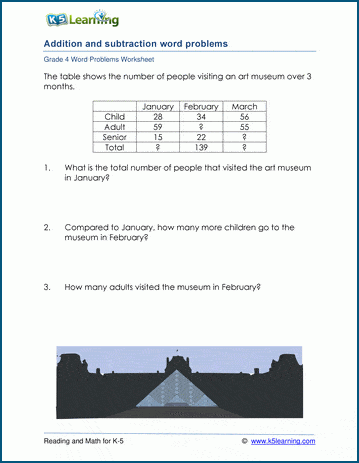# Addition & subtraction word problems

## Word problem worksheets: Mixed addition and subtraction

Below are three versions of our grade 4 math worksheet with word problems involving addition and subtraction.  Tthere may be two or three addends or subtrahends with up to 4 digits in any given problem, though generally the computations are kept relatively simple.  Some problems will include irrelevant data so that students have to read and understand the questions, rather than simply recognizing a pattern to the solutions.  The last question on each worksheet asks the student to write an equation using a variable ("X") for the unknown quantity.## More word problem worksheets

Explore all of our math word problem worksheets, from kindergarten through grade 5.

## What is K5?

K5 Learning offers reading and math worksheets, workbooks and an online reading and math program for kids in kindergarten to grade 5.  We help your children build good study habits and excel in school.